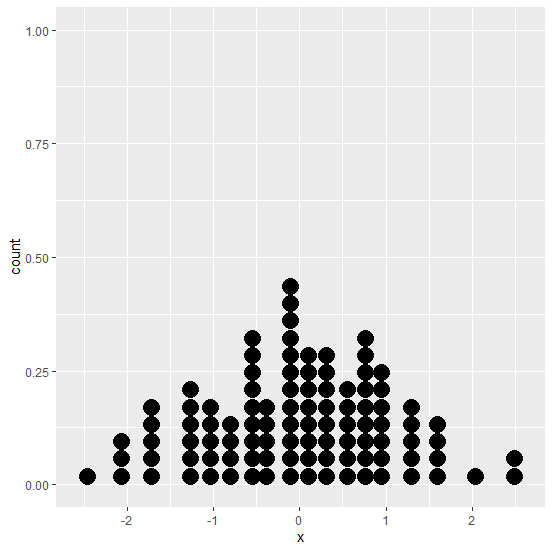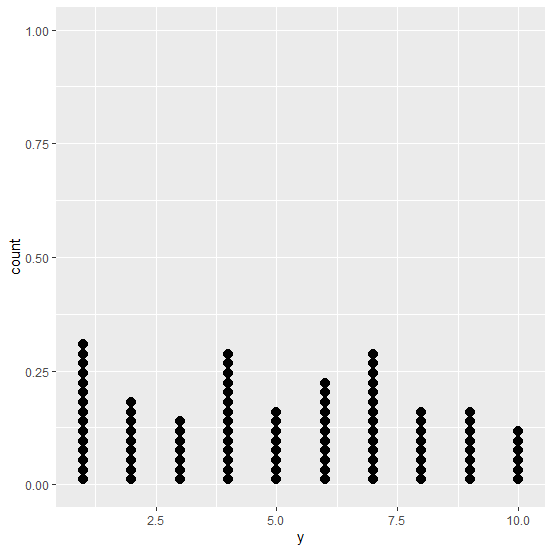# How to create a dot plot using ggplot2 in R?

A dot plot is a type of histogram that display dots instead of bars and it is created for small data sets. In ggplot2, we have geom_dotplot function to create the dot plot but we have to pass the correct binwidth which is an argument of the geom_dotplot, so that we don’t get the warning saying “Warning: Ignoring unknown parameters: bins stat_bindot() using bins = 30. Pick better value with binwidth.”

## Example

Consider the below data frame −

> x<-rnorm(100)
> df1<-data.frame(x)

> library(ggplot2)

Creating the dot plot of x −

> ggplot(df1,aes(x))+geom_dotplot(binwidth=0.2)

## OutputLet’s have a look at one more example −

> y<-sample(1:10,100,replace=TRUE)
> df2<-data.frame(y)
> ggplot(df2,aes(y))+geom_dotplot(binwidth=0.2)

## Output